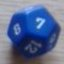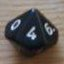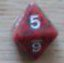Custom dice and rerolls

## Custom dice and rerolls

 Tue Jul 10 19:18:05 2018   by   danman I have a game that has custom dice. One of the sides is "+1". It adds one to your total and then you reroll the dice. So theoretically it is possible to go infinite, however improbable. I'm trying to calculate the probability of each possible result (up to a point). Here's what I want it to do:---dice := {0,1,1,2,3,3};add := 0;var := choose dice;if var = 0 then add := add+1 else var := var+add,---I have two questions. One, how do I get it to give me an output? And two, does the syntax make sense in that a 0 on the dice is the "+1", and if you don't roll a 0 you don't reroll and you add up the sum of your additives? I don't think it repeats, but I can't get foreach, repeat, or accumulate to work properly. Wed Jul 11 10:47:38 2018   by   Torben Let us start with the syntax.  The first three lines are o.k, but the last does not make sense.  The := construct defines a new variable, it does not update an existing variable, and a variable defined in a branch of an if-then-else only has scope in that branch.  Also, there is no repetition in the code you write.As I understand your problem, you want to roll your special die, and whenever it comes out 0, you roll again and add 1 to the result.  If the reroll is also a 0, you add another roll and another one, so a sequence of 0 0 2 will give a result of 2+1+1 = 4.You can use accumulate for this:`roll := accumulate x := choose {0,1,1,2,3,3} while x=0;(sum roll) + (count 0=roll)`Explanation: The first line repeats rolling the die until a non-zero value comes up, and accumulates all of the dice into `roll`. It then takes the sum of the dice (which is the only non-zero value, so we could also have written `(max roll)`) and adds the count of zeroes to this. Sat Jul 28 01:28:56 2018   by   danman I just couldn't make the syntax work in my head. The way you explained it was perfect!The special die face is essentially a (+1). Is there a way to make the special die face have different values? For each 0 currently it accumulates 1 into roll, can I just throw a "x2" in there somewhere to increment it twice per 0? Mon Aug 13 10:25:14 2018   by   Torben Hi,Sorry for the late reply, I have been on holiday without my computer, and only answered the questions that I could quickly do on my phone.Yes, you can insert something like a "×2" to get zeros to count 2.  Just change the second line to`(sum roll) + 2*(count 0=roll)`